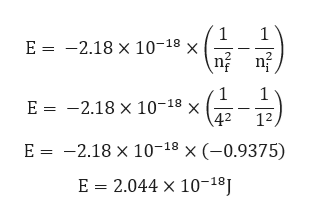# Calculate the frequency of the light emitted by a hydrogen atom during a transition of its electron from the n = 4 to the n = 1 principal energy level. Recall that for hydrogen En = –2.18 × 10–18 J(1/n2 ). (h = 6.63 × 10–34 J • s)

Question
128 views

Calculate the frequency of the light emitted by a hydrogen atom during a transition of its electron from the n = 4 to the n = 1 principal energy level. Recall that for hydrogen En = –2.18 × 10–18 J(1/n2 ). (h = 6.63 × 10–34 J • s)

check_circle

Step 1

Given:

nf = 4

ni = 1

Step 2

Energy of electromagnetic wave is given by,

Step 3

Calculation for...help_outlineImage Transcriptionclose1 2.18 x 10-18 x 1 E 1 E 2.18 x 10-18 x 42 1 12 E 2.18 x 10-18 x (-0.9375) E 2.044 x 10-18J fullscreen

### Want to see the full answer?

See Solution

#### Want to see this answer and more?

Solutions are written by subject experts who are available 24/7. Questions are typically answered within 1 hour.*

See Solution
*Response times may vary by subject and question.
Tagged in

### Chemistry